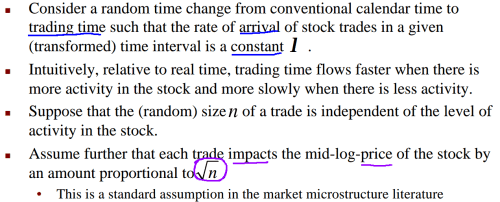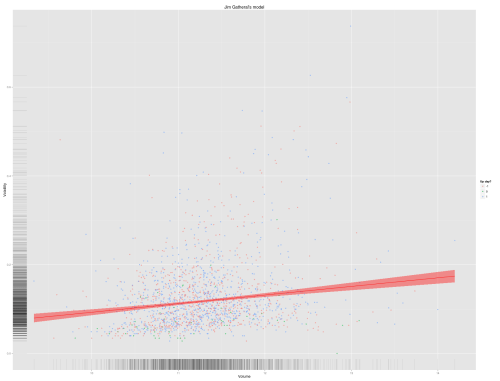Want to share your content on R-bloggers? click here if you have a blog, or here if you don't.Two interesting ideas here:

• price impact of a trade proportional to `exp( √size )`

Code follows:

```require(quantmod)
getSymbols("MER")                           #Merrill Lynch

#Gatheral's model
HiLo <- function(symbol) log( Hi(symbol) / Lo(symbol) ) **2
UpDay <- function(symbol) Cl(symbol) > Op(symbol)

#munging
mer <- merge( MER, UpDay(MER), HiLo(MER) )
mer <- data.frame(mer)
names(mer) = "UpDay"
names(mer) = "HiLo"
mer <- subset(mer, Vo(mer) > 0)          #data cleaning

#plot layers
require(ggplot2)
base <- ggplot(data=mer, aes(x=log(MER.Volume), y=HiLo, col=as.factor(UpDay)))
points <- geom_point(alpha=.5)                #no more I(.5) !
line <- geom_smooth(method='lm', col='red', fill='red')
labels <- labs(y="Volatility", x="Volume", colour="Up day?", title="Jim Gatheral's model")

#more munging
winners <- subset(mer, UpDay>0)
losers <- subset(mer, UpDay```

(This doesn’t run as is. I think you can fix that by combining the ggplot pieces differently. I just gave the pieces semantic names combing through my history.